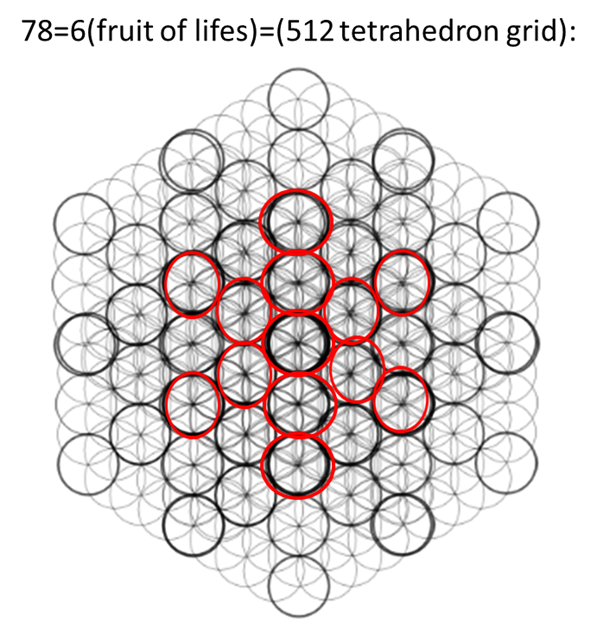top of page
Search

# 512 tetrahedron grid and infinite tetrahedron grid infinite tetractys encodes 432

432=((4:32)=(16:32)=(7:8))=78

78=(12 tetractys)=(512 tetrahedron grid)

78=56=0.833=83

83=(-0.0833)=(-1/12)=(Infinite tetractys)=(infinite tetrahedron grid)